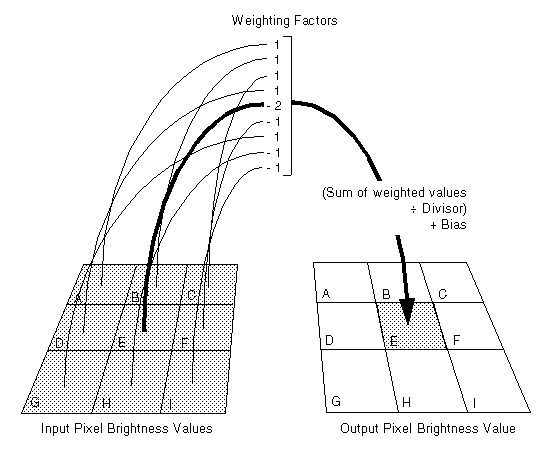﻿ Matrix Property (SpatialFilterCommand) | Leadtools.ImageProcessing.Effects | Raster, Medical, Document Help
←Select platform
In This Topic ▼

# Matrix Property

Summary
Gets or sets the array of weighting factors.
Syntax
C#
Objective-C
C++/CLI
Java
Python
``public int[] Matrix { get; set; } ``
``@property (nonatomic, copy, nullable) NSArray<NSNumber *> *matrix; ``
````public int[ getMatrix(); `
`public void setMatrix( `
`   int[] int[Value `
`); ````
````public: `
`property array<int>^ Matrix { `
`   array<int>^ get(); `
`   void set (    array<int>^ ); `
`} ````
``Matrix # get and set (SpatialFilterCommand) ``

#### Property Value

The array of weighting factors. The factors apply to pixels in the neighborhood read left to right and top to bottom in the alphabetical order shown below:Example
C#
````using Leadtools; `
`using Leadtools.Codecs; `
`using Leadtools.ImageProcessing.Effects; `
` `
` `
`public void SpatialFilterConstructorExample_S1() `
`{ `
`   // Load an image `
`   RasterCodecs codecs = new RasterCodecs(); `
`   codecs.ThrowExceptionsOnInvalidImages = true; `
` `
`   RasterImage image = codecs.Load(Path.Combine(LEAD_VARS.ImagesDir, "ImageProcessingDemo\\NaturalFruits.jpg")); `
` `
`   // Prepare the command `
`   SpatialFilterCommand command1 = new SpatialFilterCommand(SpatialFilterCommandPredefined.EmbossSouth); `
`   int[] data = command1.Matrix; `
`   SpatialFilterCommand command = new SpatialFilterCommand(); `
`   command.Divisor = command1.Divisor; `
`   command.Bias = command1.Bias; `
`   command.Matrix = data; `
`   MessageBox.Show("Array Dimension is :" + command1.Dimension.ToString()); `
`   //Apply the filter. `
`   command.Run(image); `
` `
`} `
` `
`static class LEAD_VARS `
`{ `
`   public const string ImagesDir = @"C:\LEADTOOLS22\Resources\Images"; `
`} ````
Requirements